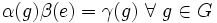# Homotopy of groups arises from a homomorphism

## Statement

Suppose$G$ and$H$ are groups, and$\alpha, \beta, \gamma$ are maps$G \to H$ such that$(\alpha,\beta,\gamma)$ is a Homotopy of magmas (?) from$G$ to$H$. In other words, for all$g_1,g_2\in G$, we have:$\! \alpha(g_1)\beta(g_2) = \gamma(g_1g_2)$

Then, there is a Homomorphism of groups (?)$\varphi:G \to H$ such that:$\! \alpha(g) = \alpha(e)\varphi(g), \beta(g) = \varphi(g)\beta(e), \gamma(g) = \alpha(e)\varphi(g)\beta(e) \ \forall \ g \in G$

where$e$ is the identity element of$G$.

In particular, since left and right multiplication maps are bijective, all the maps$\alpha,\beta,\gamma$ have the same patterns of fibers as the homomorphism$\varphi$, namely, cosets of the kernel of$\varphi$.

## Proof

Given: Groups$G,H$, a homotopy$(\alpha,\beta,\gamma):G \to H$

To prove: There is a homomorphism$\varphi:G \to H$ such that:$\! \alpha(g) = \alpha(e)\varphi(g), \beta(g) = \varphi(g)\beta(e), \gamma(g) = \alpha(e)\varphi(g)\beta(e) \ \forall \ g \in G$

Proof: Consider the defining equation of a homotopy of magmas:$\! \alpha(g_1)\beta(g_2) = \gamma(g_1g_2)$

Setting$g_1 = g, g_2 = e$ gives:$\! \alpha(g)\beta(e) = \gamma(g) \ \forall \ g \in G$

Setting$g_1 = e, g_2 = g$ gives:$\! \alpha(e) \beta(g) = \gamma(g) \ \forall \ g \in G$

Combining the above, we get:$\! \alpha(e)\beta(g) = \alpha(g)\beta(e) \ \forall \ g \in G$

This simplifies to:$\! \alpha(e)^{-1}\alpha(g) = \beta(g)\beta(e)^{-1} \ \forall \ g \in G$

Let$\varphi(g)$ be this common value. Then$\alpha(g) = \alpha(e)\varphi(g)$ and$\beta(g) = \varphi(g)\beta(e)$. Also, from the above relation, we obtain that$\gamma(g) = \alpha(g)\beta(e) = \alpha(e)\varphi(g)\beta(e)$.

It remains to show that the map$\gamma$ is a homomorphism. For this, consider the original defining relation:$\! \alpha(g_1)\beta(g_2) = \gamma(g_1g_2)$

Writing everything in terms of$\alpha(e), \beta(e), \varphi(g)$, we obtain:$\! \alpha(e) \varphi(g_1)\varphi(g_2) \beta(e) = \alpha(e)\varphi(g_1g_2)\beta(e) \ \forall \ g_1,g_2 \in G$

Canceling$\alpha(e)$ and$\beta(e)$ we obtain:$\varphi(g_1)\varphi(g_2) = \varphi(g_1g_2) \ \forall \ g_1,g_2 \in G$

Thus,$\varphi$ is a homomorphism of groups.